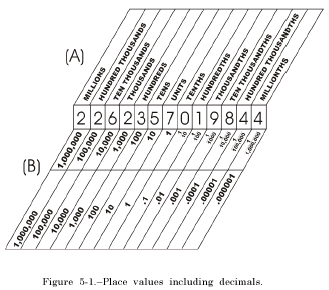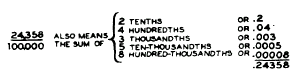Custom SearchCHAPTER 5 DECIMALS The origin and meaning of the word "decimal" were discussed in chapter 1 of this course. Also discussed in chapter 1 were the concept of place value and the use of the number ten as the base for our number system. Another term which is frequently used to denote the base of a number system is RADIX. .For example, two is the radix of the binary system and ten is the radix of the decimal system. The radix of a number system is always equal to the number of different digits used in the system. For example, the decimal system, with radix ten, has ten digits: 0 through 9. DECIMAL FRACTIONS A decimal fraction is a fraction whose denominator is 10 or some power of 10, such as 100, 1,000, or 10,000. Thus, 7/10 13/100 and 215 /1000 are decimal fractions. Decimal fractions have special characteristics that make computation much simpler than with other fractions. Decimal fractions complete our decimal system of numbers. In the study of whole numbers, we found that we could proceed to the left from the units place, tens, hundreds, thousands, and on indefinitely to any larger place value, but the development stopped with the units place. Decimal fractions complete the development so that we can proceed to the right of the units place to any smaller number indefinitely. Figure 5-l (A) shows how decimal fractions complete the system. It should be noted that as we proceed from left to right, the value of each place is one-tenth the value of the preceding place, and that the system continues uninterrupted with the decimal fractions. Figure 5-l (B) shows the system again, this time using numbers. Notice in (A) and (B) that the units place is the center of the system and that the place values proceed to the right or left of it by powers of ten. Ten on the left is balanced by tenths on the right, hundred6 by hundredths, thousands by thousandths, etc. Notice that l/10 is one place to the right of the units digit, 1/100 is two places to the right, etc. (See fig. 5-l.) If a marker is placed after the units digit, we can decide whether a decimal digit is in the tenths, hundredths, or thousandths position by counting places to the right of the marker. In some European countries, the marker is a comma; but in the English-speaking countries, the marker is the DECIMAL POINT. Thus, 3/10 is written 0.3. To write 3/100 is necessary to show that 3 is in the second place to the right of the decimal point, so a zero is inserted in the first place. Thus, 3/100 is written  Similarly, 3/1000. can be written by inserting zeros in the first two places to the right of the decimal point. Thus, 3/1000 is written 0.003. In the number 0.3, we say that 3 is in the first decimal place; in 0.03, 3 is in the second decimal place; and in 0.003, 3 is in the third decimal place. Quite frequently decimal fraction6 are simply called decimals when written in this shortened form. WRITING DECIMALS Any decimal fraction may be written in the shortened form by a simple mechanical process. Simply begin at the right-hand digit of the numerator and count off to the left as many places as there are zeros in the denominator. Place the decimal point to the left of the last digit counted. The denominator may then be disregarded. If there are not enough digits, as many place-holding zero6 as are necessary are added to the left of the left-hand digit in the numerator. Thus, is 23/10000 beginning with the digit 3, we count off four places to the left, adding two Os as we count, and place the decimal point to the extreme left. (See fig. 5-2.) Either form is read "twenty-three ten-thousandths." When a decimal fraction is written in the shortened form, there will always be as many decimal places in the shortened form as thereFigure 5-2.Conversion of a decimal fraction to shortened form.Figure 5-3.Steps in the conversion of a decimal fraction to shortened form. are zeros in the denominator of the fractional form. Figure 5-3 shows the fraction 24358/100000 and what is meant when it is changed to the short-ended form. This figure is presented to show further that each digit of a decimal fraction holds a certain position in the digit sequence and has a particular value. By the fundamental rule of fractions, it should be clear that  Writing 5/10 = 50/100 = 500/1000 the same values in the shortened way, we have 0.5 = 0.50 = 0.500. In other words, the value of a decimal is not changed by annexing zeros at the right-hand end of the number. This is not  true of whole numbers. Thus, 0.3, 0.30, and 0.300 are equal but 3, 30, and 300 are not equal. Also notice that zeros directly after the decimal point do change values. Thus 0.3 is not equal to either 0.03 or 0.003. Decimals such as 0.125 are frequently seen. Although the 0 on the left of the decimal point is not required, it is often helpful. This is particularly true in an expression such as 32 + 0.1. In this expression, the lower dot of the division symbol must not be crowded against the decimal point; the 0 serves as an effective spacer. If any doubt exists concerning the clarity of an expression such as .125, it should be written as 0.125. Practice problems. In problems 1 through 4, change the fractions to decimals. In problems 5 through 8, write the given numbers as decimals: 1. 8/100 2. 5/1000 3. 43/1000 4. 32/10000 5. Four hundredths 6. Four thousandths 7. Five hundred one ten-thousandths 8. Ninety-seven thousandths Answers: 1. 0.08 2. 0.005 3. 0.043 4. 0.0032 5. 0.04 6. 0.004 7. 0.0501 8. 0.097Integrated Publishing, Inc. - A (SDVOSB) Service Disabled Veteran Owned Small Business